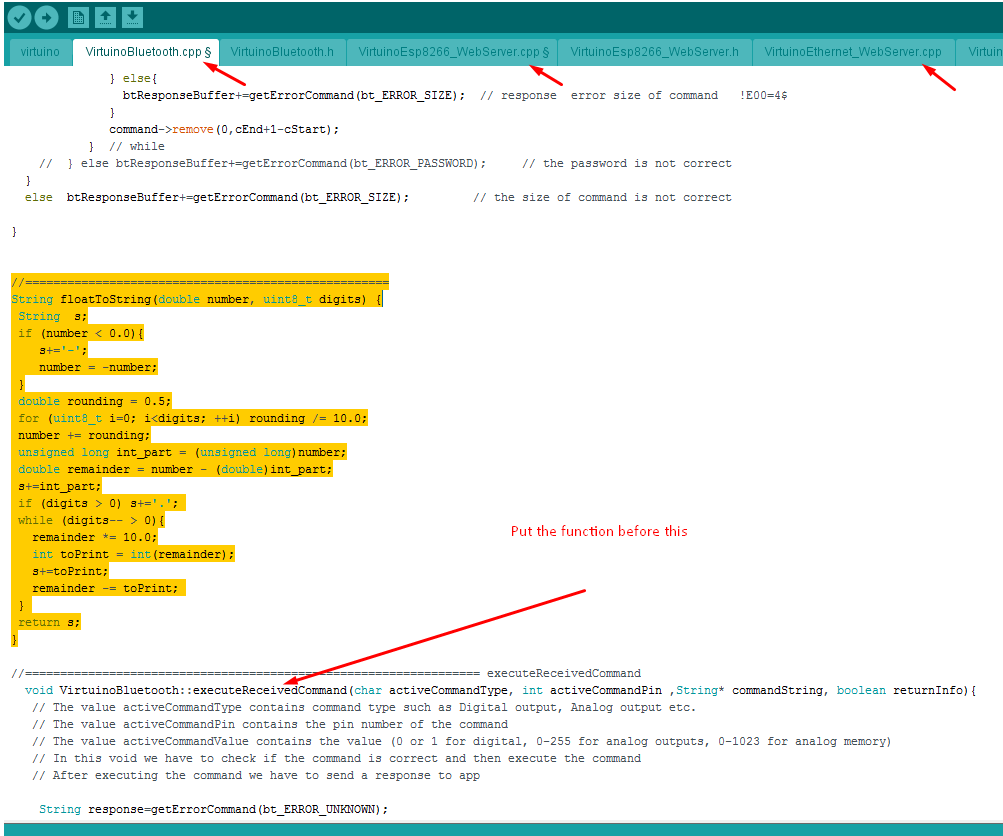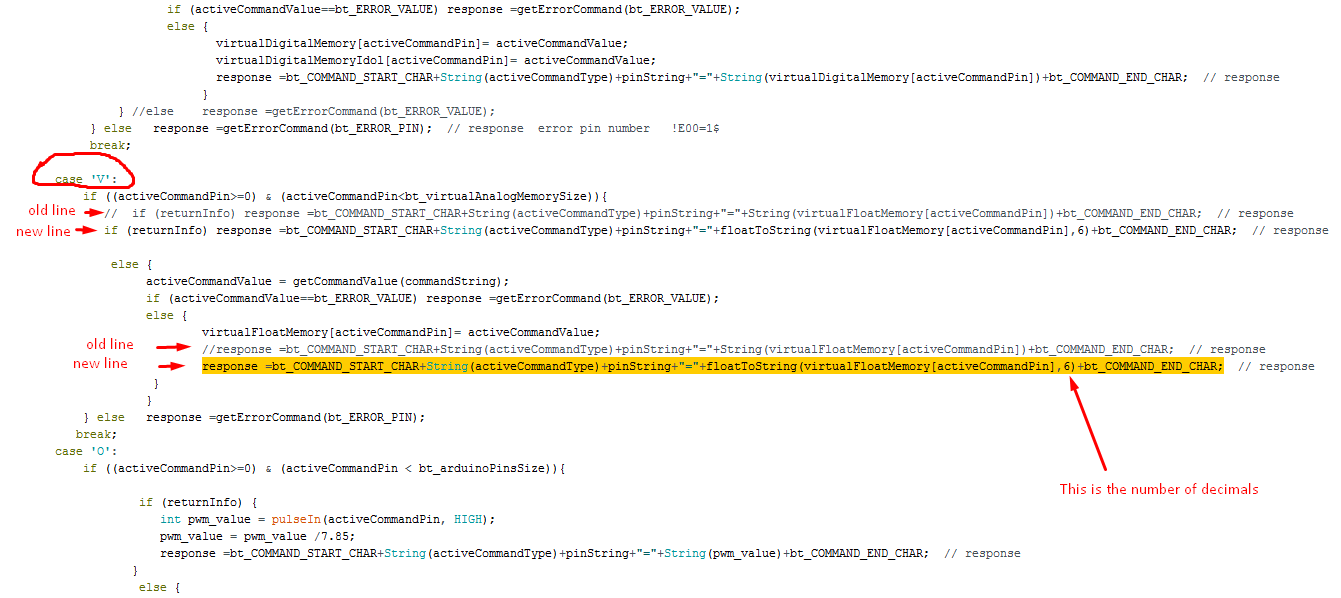How modify Virtuino library to support more than two decimal places

Step 1:

Open the virtuino library .cpp file of the library that you use (bluetooth, esp8266 or ethernet)
then put this function in the file

//====================================================
String floatToString(double number, uint8_t digits) {
String  s;
if (number < 0.0){
s+='-';
number = -number;
}
double rounding = 0.5;
for (uint8_t i=0; i<digits; ++i) rounding /= 10.0;
number += rounding;
unsigned long int_part = (unsigned long)number;
double remainder = number - (double)int_part;
s+=int_part;
if (digits > 0) s+='.';
while (digits-- > 0){
remainder *= 10.0;
int toPrint = int(remainder);
s+=toPrint;
remainder -= toPrint;
}
return s;
}Step 2:

Make these changes to this part of the codedisable the old line as the  example and put the following new line

response =bt_COMMAND_START_CHAR+String(activeCommandType)+pinString+"="+floatToString(virtualFloatMemory[activeCommandPin],6)+bt_COMMAND_END_CHAR;

You can't make these changes to all .cpp files (bluetooth, esp8266,ethernet) but only on one each time

Then save the file and compile.